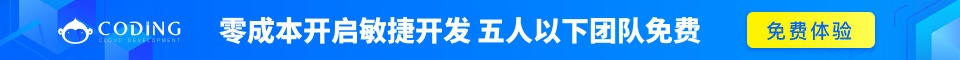# Python 输入和输出

## 输出格式美化

Python两种输出值的方式: 表达式语句 和 print() 函数。(第三种方式是使用文件对象的 write() 方法; 标准输出文件可以用 sys.stdout 引用。)

str() 函数返回一个用户易读的表达形式。

repr() 产生一个解释器易读的表达形式。

### 例如

```>>> s = 'Hello, world.'
>>> str(s)
'Hello, world.'
>>> repr(s)
"'Hello, world.'"
>>> str(1/7)
'0.14285714285714285'
>>> x = 10 * 3.25
>>> y = 200 * 200
>>> s = 'The value of x is ' + repr(x) + ', and y is ' + repr(y) + '...'
>>> print(s)
The value of x is 32.5, and y is 40000...
>>> # The repr() of a string adds string quotes and backslashes:
... hello = 'hello, world\n'
>>> hellos = repr(hello)
>>> print(hellos)
'hello, world\n'
>>> # The argument to repr() may be any Python object:
... repr((x, y, ('spam', 'eggs')))
"(32.5, 40000, ('spam', 'eggs'))"
```

```>>> for x in range(1, 11):
...     print(repr(x).rjust(2), repr(x*x).rjust(3), end=' ')
...     # Note use of 'end' on previous line 注意前一行 'end' 的使用
...     print(repr(x*x*x).rjust(4))
...
1   1    1
2   4    8
3   9   27
4  16   64
5  25  125
6  36  216
7  49  343
8  64  512
9  81  729
10 100 1000

>>> for x in range(1, 11):
...     print('{0:2d} {1:3d} {2:4d}'.format(x, x*x, x*x*x))
...
1   1    1
2   4    8
3   9   27
4  16   64
5  25  125
6  36  216
7  49  343
8  64  512
9  81  729
10 100 1000
```

```>>> '12'.zfill(5)
'00012'
>>> '-3.14'.zfill(7)
'-003.14'
>>> '3.14159265359'.zfill(5)
'3.14159265359'
```

str.format() 的基本使用如下:

```>>> print('We are the {} who say "{}!"'.format('knights', 'Ni'))
We are the knights who say "Ni!"
```

```>>> print('{0} and {1}'.format('spam', 'eggs'))
spam and eggs
>>> print('{1} and {0}'.format('spam', 'eggs'))
eggs and spam
```

```>>> print('This {food} is {adjective}.'.format(
This spam is absolutely horrible.
```

```>>> print('The story of {0}, {1}, and {other}.'.format('Bill', 'Manfred',
other='Georg'))
The story of Bill, Manfred, and Georg.
```
'!a' (使用 ascii()), '!s' (使用 str()) 和 '!r' (使用 repr()) 可以用于在格式化某个值之前对其进行转化:
```>>> import math
>>> print('The value of PI is approximately {}.'.format(math.pi))
The value of PI is approximately 3.14159265359.
>>> print('The value of PI is approximately {!r}.'.format(math.pi))
The value of PI is approximately 3.141592653589793.
```

```>>> import math
>>> print('The value of PI is approximately {0:.3f}.'.format(math.pi))
The value of PI is approximately 3.142.
```

```>>> table = {'Sjoerd': 4127, 'Jack': 4098, 'Dcab': 7678}
>>> for name, phone in table.items():
...     print('{0:10} ==> {1:10d}'.format(name, phone))
...
Jack       ==>       4098
Dcab       ==>       7678
Sjoerd     ==>       4127
```

```>>> table = {'Sjoerd': 4127, 'Jack': 4098, 'Dcab': 8637678}
>>> print('Jack: {0[Jack]:d}; Sjoerd: {0[Sjoerd]:d}; '
'Dcab: {0[Dcab]:d}'.format(table))
Jack: 4098; Sjoerd: 4127; Dcab: 8637678
```

```>>> table = {'Sjoerd': 4127, 'Jack': 4098, 'Dcab': 8637678}
>>> print('Jack: {Jack:d}; Sjoerd: {Sjoerd:d}; Dcab: {Dcab:d}'.format(**table))
Jack: 4098; Sjoerd: 4127; Dcab: 8637678
```

## 旧式字符串格式化

% 操作符也可以实现字符串格式化。 它将左边的参数作为类似 sprintf() 式的格式化字符串, 而将右边的代入, 然后返回格式化后的字符串. 例如:

```>>> import math
>>> print('The value of PI is approximately %5.3f.' % math.pi)
The value of PI is approximately 3.142.
```

## 读和写文件

open() 将会返回一个 file 对象，基本语法格式如下:

```open(filename, mode)
```

```>>> f = open('/tmp/workfile', 'w')
```
• 第一个参数为要打开的文件名。
• 第二个参数描述文件如何使用的字符。 mode 可以是 'r' 如果文件只读, 'w' 只用于写 (如果存在同名文件则将被删除), 和 'a' 用于追加文件内容; 所写的任何数据都会被自动增加到末尾. 'r+' 同时用于读写。 mode 参数是可选的; 'r' 将是默认值。

## 文件对象的方法

size 是一个可选的数字类型的参数。 当 size 被忽略了或者为负, 那么该文件的所有内容都将被读取并且返回。

```>>> f.read()
'This is the entire file.\n'
''
```

```>>> f.readline()
'This is the first line of the file.\n'
'Second line of the file\n'
''
```

```>>> f.readlines()
['This is the first line of the file.\n', 'Second line of the file\n']
```

```>>> for line in f:
...     print(line, end='')
...
This is the first line of the file.
Second line of the file
```

### f.write()

f.write(string) 将 string 写入到文件中, 然后返回写入的字符数。

```>>> f.write('This is a test\n')
15
```

```>>> value = ('the answer', 42)
>>> s = str(value)
>>> f.write(s)
18
```

### f.tell()

f.tell() 返回文件对象当前所处的位置, 它是从文件开头开始算起的字节数。

### f.seek()

from_what值为0时表示文件的开始，它也可以省略，缺省是0即文件开头。下面给出一个完整的例子：

```>>> f = open('/tmp/workfile', 'rb+')
>>> f.write(b'0123456789abcdef')
16
>>> f.seek(5)     # 移动到文件的第六个字节
5
b'5'
>>> f.seek(-3, 2) # 移动到文件的倒数第三字节
13
b'd'
```

### f.close()

```>>> f.close()
Traceback (most recent call last):
File "<stdin>", line 1, in ?
ValueError: I/O operation on closed file
<pre>
<p>

<pre>
>>> with open('/tmp/workfile', 'r') as f:
>>> f.closed
True
```

## pickle 模块

python的pickle模块实现了基本的数据序列和反序列化。

```pickle.dump(obj, file, [,protocol])
```

```x = pickle.load(file)
```

```#使用pickle模块将数据对象保存到文件

import pickle

data1 = {'a': [1, 2.0, 3, 4+6j],
'b': ('string', u'Unicode string'),
'c': None}

selfref_list = [1, 2, 3]
selfref_list.append(selfref_list)

output = open('data.pkl', 'wb')

# Pickle dictionary using protocol 0.
pickle.dump(data1, output)

# Pickle the list using the highest protocol available.
pickle.dump(selfref_list, output, -1)

output.close()
```

```#使用pickle模块从文件中重构python对象

import pprint, pickle

pkl_file = open('data.pkl', 'rb')